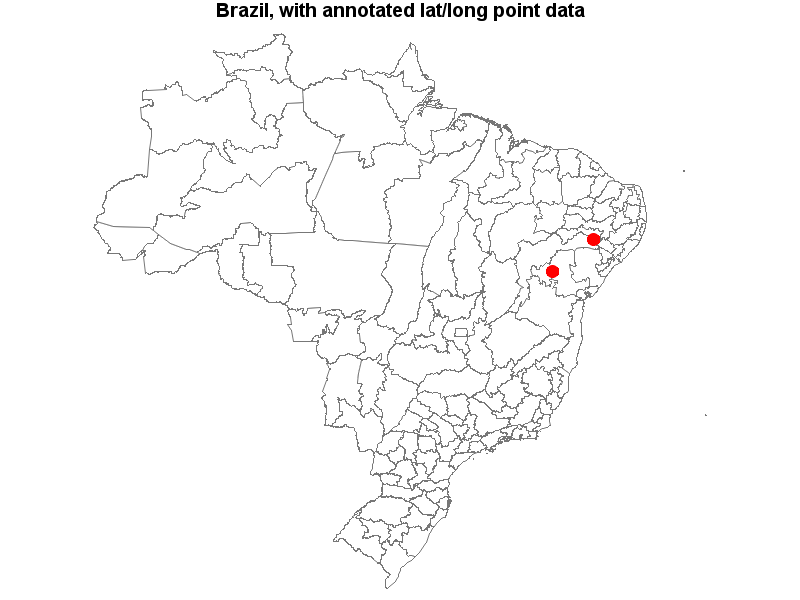## Latitude and Longitude in proc gmap

I have this map with the Brazilian states:

``````data estados;
set maps.brazil;
run;
proc sort data = work.estados nodupkey;by ID;run;
xsys='2'; ysys='2'; hsys='3'; when='a';
function='pie'; style='psolid'; rotate=360; size=1; color='red';
run;

pattern1 v=s c='white';

id id;
choro segment / levels=1 nolegend coutline=gray88;
run;``````

the `"anno=work.estados"` mark some states on the map.

I have a dataset with coordinates (latitude and longitude), someone know how i show a marker for each coordinate?

2 REPLIES 2

## Re: Latitude and Longitude in proc gmap

The Maps.Brazil data set contains Lat and Long variables. So if your annotate data does as well and have the same names you may be able to use the LATLON option on Proc Gmap to use those instead of the X Y variables for plotting.

```proc gmap map=maps.brazil anno=work.estados
LATLON
;
id id;
choro segment / levels=1 nolegend coutline=gray88;
run;```

## Re: Latitude and Longitude in proc gmap

If you're working with lat/long point data, I would recommend projecting the map, and then projecting the point data (using the exact same parameters as were used to project the map). You could work with unprojected lat/long values, but having the code in place to apply any projection you want really adds a lot of flexibility (and is the best-practice way to go).

Here's an example:

data my_map; set mapsgfk.brazil (where=(density<=3) drop=x y resolution);
run;

proc gproject data=my_map out=my_map latlong eastlong degrees parmout=projparm;
id id;
run;

data point_data;
input lat long;
datalines;
-11.3994052 -41.2813161
-9.0266125 -38.4466716
;
run;

proc gproject data=point_data out=point_data latlong eastlong degrees parmin=projparm parmentry=my_map;
id;
run;

data anno_point_data; set point_data;
xsys='2'; ysys='2'; hsys='3'; when='a';
function='pie'; style='psolid'; rotate=360; size=1; color='red';
run;

pattern1 v=s c=white;

title "Brazil, with annotated lat/long point data";
proc gmap map=my_map data=my_map anno=anno_point_data;
id id;
choro segment / levels=1 nolegend coutline=gray77;
run;Discussion stats
• 2 replies
• 591 views
• 0 likes
• 3 in conversation